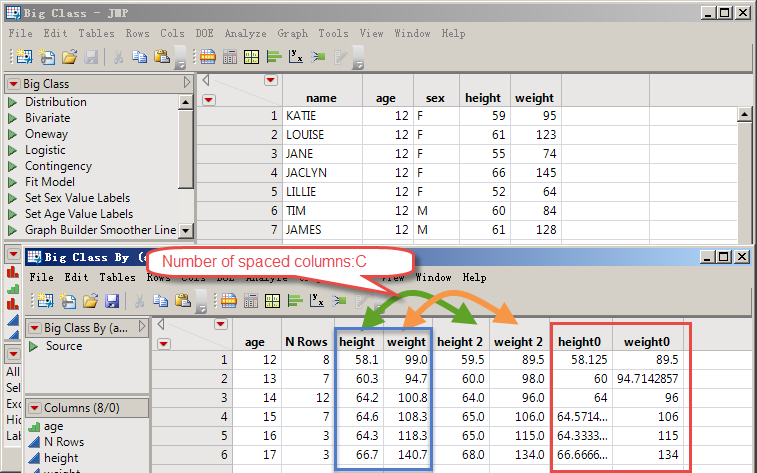Choose Language Hide Translation Bar
Highlighted

## How do use JSL to quickly compare two sets of range columns in order to get the minimum of each column?

The title is a bit hard to understand.

Take this big class as an example:
1. First, summarize the average values of "height" and "weight" by age.
2. Then summarize the median of "height" and "weight" by age.
3. Then compare the mean and median of "height" and take their minimum.
Finally, compare the mean and median of "body weight" and take their minimum.I'll use the following code and finally loop through to get the minimum.
Is there any good code that minimizes this comparison?

``````dt = Open( "\$SAMPLE_DATA/Big Class.jmp" );
d1 = dt << Summary(
Group( :age ),
Mean( :height ),
Mean( :weight ),
Median( :height ),
Median( :weight ),
Freq( "None" ),
Weight( "None" ),
Link to original data table( 0 ),
statistics column name format( "column" )
);
c = N Col( d1 ) / 2 - 1;
For( i = 1, i <= c, i++,
ca = Column( i + 2 ) << Get Name || "0";
d1 << New Column( ca, formula( Min( As Column( 2 + i ), As Column( 2 + c + i ) ) ) );
d1 << run formulas;
Column( ca ) << deleteFormula;

);``````

Thanks!

1 ACCEPTED SOLUTION

Accepted Solutions
Highlighted

## Re: How do use JSL to quickly compare two sets of range columns in order to get the minimum of each column?

Here is a possible quicker solution for you

``````Names Default To Here( 1 );
dtOrig = open("\$SAMPLE_DATA/semiconductor capability.jmp");
colNames = dtOrig << get column names(continuous);
dt = dtOrig << Summary(
Group( :SITE ),
mean(colnames),median(colnames),
);

half = N Col( dt ) / 2 - 1;
For( i = 3, i <= half, i++,
mat = dt[0, i];
mat = mat || dt[0, i + half];
v = Transpose( V Min( Transpose( mat ) ) );

dt << New Column( "Min" || Substr( Column( dt, i ) << get name, Contains( Column( dt, i ) << get name, "(" ) ),
set values( v )
);
);``````
Jim
4 REPLIES 4
Highlighted

## Re: How do use JSL to quickly compare two sets of range columns in order to get the minimum of each column?

This looping method is slow when the number of columns and rows that need to be summarized for comparison is large.
Is the matrix method faster?Or there are other good ways.

Thanks Experts!

Highlighted

## Re: How do use JSL to quickly compare two sets of range columns in order to get the minimum of each column?

And it's inefficient:
Min( As Column( 2 + i ), As Column( 2 + c + i ) ) )
Highlighted

## Re: How do use JSL to quickly compare two sets of range columns in order to get the minimum of each column?

Here is a possible quicker solution for you

``````Names Default To Here( 1 );
dtOrig = open("\$SAMPLE_DATA/semiconductor capability.jmp");
colNames = dtOrig << get column names(continuous);
dt = dtOrig << Summary(
Group( :SITE ),
mean(colnames),median(colnames),
);

half = N Col( dt ) / 2 - 1;
For( i = 3, i <= half, i++,
mat = dt[0, i];
mat = mat || dt[0, i + half];
v = Transpose( V Min( Transpose( mat ) ) );

dt << New Column( "Min" || Substr( Column( dt, i ) << get name, Contains( Column( dt, i ) << get name, "(" ) ),
set values( v )
);
);``````
Jim
Highlighted

Thank Jim!

Article Labels